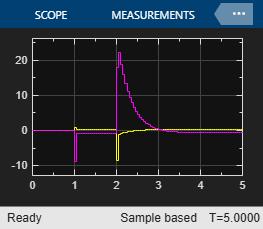Main Content

# Aircraft with Unstable Poles

This example shows how to control an unstable aircraft with saturating actuators.

For an example that controls the same plant using an explicit MPC controller, see Explicit MPC Control of an Aircraft with Unstable Poles.

### Define Aircraft Model

The linear open-loop dynamic model of the aircraft has the following state-space matrices:

```A = [-0.0151 -60.5651 0 -32.174; -0.0001 -1.3411 0.9929 0; 0.00018 43.2541 -0.86939 0; 0 0 1 0]; B = [-2.516 -13.136; -0.1689 -0.2514; -17.251 -1.5766; 0 0]; C = [0 1 0 0; 0 0 0 1]; D = [0 0; 0 0]; ```

Create the plant, and specify the initial states as zero.

```plant = ss(A,B,C,D); x0 = zeros(4,1); ```

The manipulated variables are the elevator and flaperon angles. The attack and pitch angles are measured outputs to be regulated.

The open-loop response of the system is unstable.

```pole(plant) ```
```ans = -7.6636 + 0.0000i 5.4530 + 0.0000i -0.0075 + 0.0556i -0.0075 - 0.0556i ```

### Specify Controller Constraints

Both manipulated variables are constrained between +/- 25 degrees. Since the plant inputs and outputs are of different orders of magnitude, you also use scale factors to facilitate MPC tuning. Typical choices of scale factor are the upper/lower limit or the operating range.

```MV = struct('Min',{-25,-25},'Max',{25,25},'ScaleFactor',{50,50}); ```

Both plant outputs have constraints to limit undershoots at the first prediction horizon. You also specify scale factors for outputs.

```OV = struct('Min',{[-0.5;-Inf],[-100;-Inf]},... 'Max',{[0.5;Inf],[100;Inf]},... 'ScaleFactor',{1,200}); ```

### Specify Controller Tuning Weights

The control task is to get zero offset for piecewise-constant references, while avoiding instability due to input saturation. Because both MV and OV variables are already scaled in MPC controller, MPC weights are dimensionless and applied to the scaled MV and OV values. In this example, you penalize the two outputs equally with the same OV weights.

```Weights = struct('MV',[0 0],'MVRate',[0.1 0.1],'OV',[10 10]); ```

### Create MPC Controller

Create an MPC controller with the specified plant model, sample time, and horizons.

```Ts = 0.05; % Sample time p = 10; % Prediction horizon m = 2; % Control horizon mpcobj = mpc(plant,Ts,p,m,Weights,MV,OV); ```

### Simulate Using Simulink®

To run this example, Simulink® is required.

```if ~mpcchecktoolboxinstalled('simulink') disp('Simulink(R) is required to run this example.') return end ```

Simulate closed-loop control of the linear plant model in Simulink. To do so, for the MPC Controller block, set the MPC Controller property to `mpcobj`.

```mdl = 'mpc_aircraft'; open_system(mdl) sim(mdl) ```
```-->Converting model to discrete time. -->Assuming output disturbance added to measured output channel #1 is integrated white noise. -->Assuming output disturbance added to measured output channel #2 is integrated white noise. -->The "Model.Noise" property of the "mpc" object is empty. Assuming white noise on each measured output channel. ```The closed-loop response shows good setpoint tracking performance.

### References

 P. Kapasouris, M. Athans, and G. Stein, "Design of feedback control systems for unstable plants with saturating actuators", Proc. IFAC Symp. on Nonlinear Control System Design, Pergamon Press, pp.302--307, 1990

 A. Bemporad, A. Casavola, and E. Mosca, "Nonlinear control of constrained linear systems via predictive reference management", IEEE® Trans. Automatic Control, vol. AC-42, no. 3, pp. 340-349, 1997.

```bdclose(mdl) ```

## Support

#### Implementing an Adaptive Cruise Controller with Simulink

Download technical paper